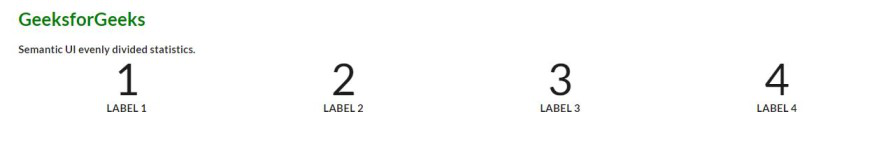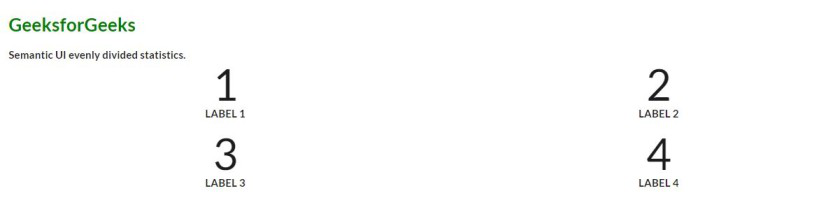GeeksforGeeks App
Open AppBrowser
Continue

# Semantic-UI Statistics Evenly Divided Variant

Semantic UI is an open-source framework that offers a variety of components to make your UI more meaningful. One of them is “Statistics”. The statistics component is used to magnify particular data for your website. Users can also position the statistics as per their requirements. Let us see this statistics variation.

Semantic-UI Statistics offers us 2 types o statistics with 6 types of variation for those two Horizontal, Coloured, Inverted, Evenly Divided, Floated, Clearing. In this article, we will know about the Semantic-UI Statistics Evenly Divided Variant.

Statistics Evenly Divided Variation offers to decide how many statistics you want to show in a row. A web page can contain a bunch of statistics. You can divide them with a precise number using this variation. We will create a UI showing the statistics evenly divided variation.

Semantic-UI Statistics Evenly Divided Variant Class:

• *number: This is a numeric value holder that divides the container in equal width of the component.

Syntax:

```<div class="ui *number statistics">
<div class="statistic">
....
</div>
<div class="statistic">
....
</div>
....
</div>```

Below example illustrate the Semantic-UI Statistics Evenly Divided Variant:

Example 1: This example demonstrates evenly divided statistics using the statistics class along with four statistics in a row.

## HTML

 ```<``html``>`` ` `<``head``>``    ``<``link` `href``=``"https://cdnjs.cloudflare.com/ajax/libs/semantic-ui/2.4.1/semantic.min.css"` `          ``rel``=``"stylesheet"` `/>```` ` `<``body``>``    ``<``div` `class``=``"ui container"``>``        ``<``h2` `style``=``"color:green"``>GeeksforGeeks``        ``<``b``>``            ``<``p``>Semantic UI evenly divided statistics.``        ````        ``<``div` `class``=``"ui four statistics"``>``            ``<``div` `class``=``"statistic"``>``                ``<``div` `class``=``"value"``>``                    ``1``                ````                ``<``div` `class``=``"label"``>``                    ``Label 1``                ````            ````            ``<``div` `class``=``"statistic"``>``                ``<``div` `class``=``"value"``>``                    ``2``                ````                ``<``div` `class``=``"label"``>``                    ``Label 2``                ````            ````            ``<``div` `class``=``"statistic"``>``                ``<``div` `class``=``"value"``>``                    ``3``                ````                ``<``div` `class``=``"label"``>``                    ``Label 3``                ````            ````            ``<``div` `class``=``"statistic"``>``                ``<``div` `class``=``"value"``>``                    ``4``                ````                ``<``div` `class``=``"label"``>``                    ``Label 4``                ````            ````        ````    `````` ` ``

Output:Semantic-UI Statistics Evenly Divided Variant

Example 2: This example demonstrates evenly divided statistics using the statistics class along with two statistics in a row.

## HTML

 ```<``html``>`` ` `<``head``>``    ``<``link` `href``=``"https://cdnjs.cloudflare.com/ajax/libs/semantic-ui/2.4.1/semantic.min.css"` `          ``rel``=``"stylesheet"` `/>```` ` `<``body``>``    ``<``div` `class``=``"ui container"``>``        ``<``h2` `style``=``"color:green"``>GeeksforGeeks``        ``<``b``>``            ``<``p``>Semantic UI evenly divided statistics.``        ````        ``<``div` `class``=``"ui two statistics"``>``            ``<``div` `class``=``"statistic"``>``                ``<``div` `class``=``"value"``>``                    ``1``                ````                ``<``div` `class``=``"label"``>``                    ``Label 1``                ````            ````            ``<``div` `class``=``"statistic"``>``                ``<``div` `class``=``"value"``>``                    ``2``                ````                ``<``div` `class``=``"label"``>``                    ``Label 2``                ````            ````            ``<``div` `class``=``"statistic"``>``                ``<``div` `class``=``"value"``>``                    ``3``                ````                ``<``div` `class``=``"label"``>``                    ``Label 3``                ````            ````            ``<``div` `class``=``"statistic"``>``                ``<``div` `class``=``"value"``>``                    ``4``                ````                ``<``div` `class``=``"label"``>``                    ``Label 4``                ````            ````        ````    `````` ` ``

Output:Semantic-UI Statistics Evenly Divided Variant

My Personal Notes arrow_drop_up
Related Tutorials